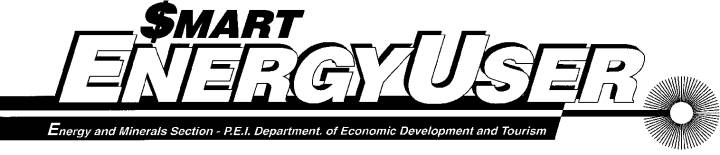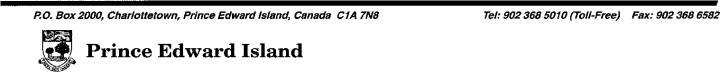October  1996                                                                                                                                  Volume 2   Issue 17 Calculating What it Costs to Run a Motor     Much of the electrical energy consumed in a typical production operation flows through large-horsepower electric motors.  Because of this, it makes sense to gather data on your large motors and figure out how much it costs to run each one.  The data gathered is valuable for other purposes too.  It allows you to identify motors that are underloaded (and thus costly to operate) or overloaded (and at risk of burnout).  This data is also extremely valuable to have on hand when a motor fails and you must quickly decide whether to repair it or replace it.       With some types of electrical load — lighting loads for example — calculating the monthly kWh consumption is straightforward.  Whenever these loads are on, they draw a constant amount of power, and nameplate amps and watts normally reflect actual operating conditions.  The monthly kWh consumption is simply the unit’s rated power in kW times the number of hours per month that the load is on.      With motors, the situation is more complex.  The motor nameplate may say Horsepower: 20, Volts: 230, and Amps: 49.0, but the Horsepower and Amps figures on the nameplate are just the motor’s maximum ratings.  They don’t indicate what the operating values really are in your situation.  The actual current that a motor draws from the line, the power it demands, and the energy it consumes, all relate to the amount of mechanical power that the motor is called upon to deliver through its output shaft.  If the motor actually delivers 20 horsepower (hp) of mechanical power, then the motor will draw 49 amps.  But under light load, the current drawn by such a motor will be significantly less.    DOING A ROUGH CALCULATION    If the load on a motor is fairly constant, and if you have access to a voltmeter and clip-on ammeter, it is easy to arrive at rough kVA and kWh figures.  For a 3-phase motor the procedure is: 1.      Measure all three phase-to-phase voltages, and average them.  In our 20 hp motor example, let’s say we measure 226, 230 and 234 volts, giving an average of 230 volts. 2.      Measure the current in each of the phase wires going to the motor, and average them.  We measure 41, 42, and 43 amps, giving an average of 42 amps.  3.      Calculate the kVA: kVA = 0.00173 × volts × amps In our example: kVA = 0.00173 × 230 × 42 kVA = 16.7 4.      Calculate the kW: kW = kVA × PF × 0.01       (where PF is the power factor in %) Unfortunately, a voltmeter and clip-on ammeter won’t tell us what the PF is.  Still, even if we don’t have equipment to measure PF directly, we can estimate it: First, calculate % Max Amps — actual motor current as a percentage of rated full load current.                 100 × measured motor amps % Max Amps = ——————————————                 max. rated motor amps In our example, % Max Amps = 100 × 42 ÷ 49 = 86       Approximate Motor Power Factor         at Various Input Currents% Max Amps (Input Amps as % of Max Rated Amps)   Second, from the graph above, estimate the motor PF.  Do this by choosing the motor power curve closest in value to the rating of your motor.  Then, following along that curve, see what the PF is at the % Max Amps value you calculated above.  In our example, following the 20 hp curve to a % Max Amps value of 86 gives a PF of approximately 76 per cent. Third, calculate the input power in kW.  kW = kVA × PF × 0.01       (PF in %) And for our example: kW = 16.7 × 76 × 0.01 = 12.7 5.      Calculate monthly kWh:       kWh = kW × hours of operation per month Let’s assume that our motor operates 333 hours per month.   kWh = 12.7 × 333 = 4230 6.      Calculate the cost. First, figure out how much each kWh costs your firm by taking a recent electricity bill and dividing the total dollar amount (including taxes) by the total number of kWh consumed during the billing period.  Second, multiply this number times the kWh value calculated in Step 5 above.  This will give the approximate monthly cost of running the motor — including that motor’s share of demand charges and taxes as well as its kWh consumption.  In our example, if the average cost of a kWh is 11.2 cents, then the cost of running this 20 hp motor would be 4230 × 0.112, or \$474 a month Note:  If you see a way to reduce the load on the motor or reduce its running time, you may want to know how much you will save.  To perform an accurate cost calculation requires taking into account the multiple “block” structure of some electricity rates.  For help with this, contact Mike Proud or Ron Estabrooks at 368-5010 (toll free). UNDERLOADED AND OVERLOADED MOTORS     Another reason to make motor measurements is to ensure that the motors in your plant are properly sized for the tasks they perform.  Motors that are built into commercial equipment tend to be properly sized, but other motors may not be.  For one thing, motors are often specified to have more horsepower than is actually required.  These underloaded motors cost more to run than properly loaded ones because they operate on a less efficient part of their load curve.  At the other extreme, some motors are called upon to deliver more power than they are designed to deliver.  They will do this for a while, but they overheat and eventually fail.  A MORE ACCURATE ASSESSMENT     In some applications the load on a motor is not constant, but varies greatly (and perhaps unpredictably) from moment to moment and hour to hour.  Here, longer-term data gathering is needed, using a device known as a recording wattmeter.  The Energy and Minerals Section has one of these units, and if you think it might shed light on your situation, call Mike Proud or Ron Estabrooks at 368-5010 (toll free).